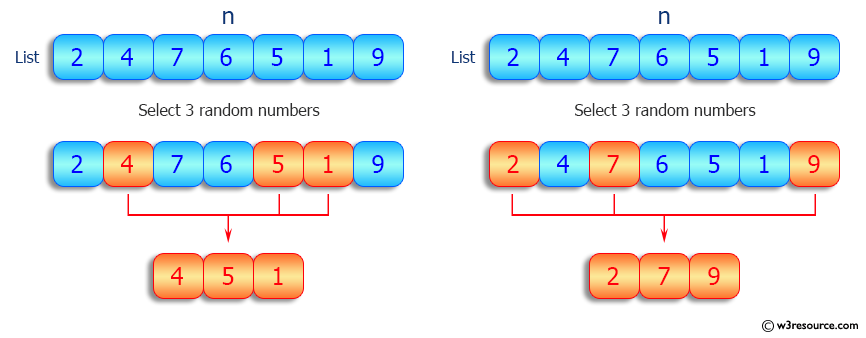﻿ Python: Extract a given number of randomly selected elements from a given list - w3resource# Python: Extract a given number of randomly selected elements from a given list

## Python List: Exercise - 81 with Solution

Write a Python program to extract a given number of randomly selected elements from a given list.

Sample Solution:

Python Code:

``````import random
def random_select_nums(n_list, n):
return random.sample(n_list, n)
n_list = [1,1,2,3,4,4,5,1]
print("Original list:")
print(n_list)
selec_nums = 3
result = random_select_nums(n_list, selec_nums)
print("\nSelected 3 random numbers of the above list:")
print(result)
```
```

Sample Output:

```Original list:
[1, 1, 2, 3, 4, 4, 5, 1]

Selected 3 random numbers of the above list:
[4, 4, 1]
```

Pictorial Presentation:Flowchart:## Visualize Python code execution:

The following tool visualize what the computer is doing step-by-step as it executes the said program:

Python Code Editor:

Have another way to solve this solution? Contribute your code (and comments) through Disqus.

What is the difficulty level of this exercise?

Test your Python skills with w3resource's quiz

﻿

## Python: Tips of the Day

Floor Division:

When we speak of division we normally mean (/) float division operator, this will give a precise result in float format with decimals.

For a rounded integer result there is (//) floor division operator in Python. Floor division will only give integer results that are round numbers.

```print(1000 // 300)
print(1000 / 300)```

Output:

```3
3.3333333333333335```